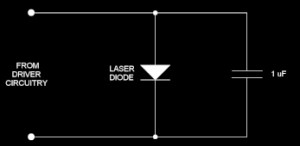ESD polarity terminology used on this web site

The term “positive-ESD” is used to mean electrostatic discharge (ESD) whose voltage polarity would tend to forward-bias a laser diode. “Negative-ESD,” means ESD whose voltage polarity would tend to reverse-bias a laser diode.

 Review of ESD approaches START PREV CURRENT NEXT END Simple capacitor

Review of ESD approaches

# Simple capacitor used as an ESD protection meansThe figure to the right shows a similar scheme that is currently in use – one in which a capacitor is placed across a laser diode. In this application, typical capacitor values range from several hundred nanofarad to several microfarad.

At first glance, a 1-microfarad capacitor would appear to be sufficient to limit a 15,000-volt ESD event from exceeding the maximum reverse bias voltage of 2.0 volts and similar forward-bias limits. However, in real life, there are no capacitors known to exist that have purely capacitive characteristics.

All known real-world electrical components have parasitic properties. Small capacitors can be modeled by the nominal capacitance in series with a parasitic resistance in series with a parasitic inductance.

As discussed, an ESD event occurs within the range of a below one nanosecond up to a few tens of nanoseconds; thus, the frequency-domain equivalent of this is around 20 MHz up to 1 GHz. Therefore, the impedance of the capacitor would need to be less than 44 milliohms between around 20 MHz and 1 GHz in order to be effective at protecting the laser diode.

Common 1-microfarad electrolytic capacitors have an equivalent series resistance of 1 ohm, and an equivalent series inductance of around 15 nanohenry. This combination clearly gives an impedance greater than 44 milliohms. And although the best 1-microfarad tantalum capacitors have an equivalent series resistance that can approach 50 milliohms, their equivalent series inductance is usually at least 1 nanohenry, which gives an impedance over 6 ohms at 1 GHz. It is not known whether a capacitor actually exists whose impedance is 44 milliohms within the frequency range of interest.

However, even if a perfect capacitor were used that would be effective at protecting the laser diode against ESD, such a capacitance makes direct modulation of a laser diode increasingly difficult, especially at high modulation frequencies. Thus, there are clear drawbacks to this simple capacitive approach.

Moreover, other studies have shown how ESD can destroy ceramic capacitors. Such a study can be found here: http://www.ce-mag.com/archive/01/Spring/Lee.html